# Quantum computing Alex Karassev Quantum Computer Quantum computer

• Slides: 30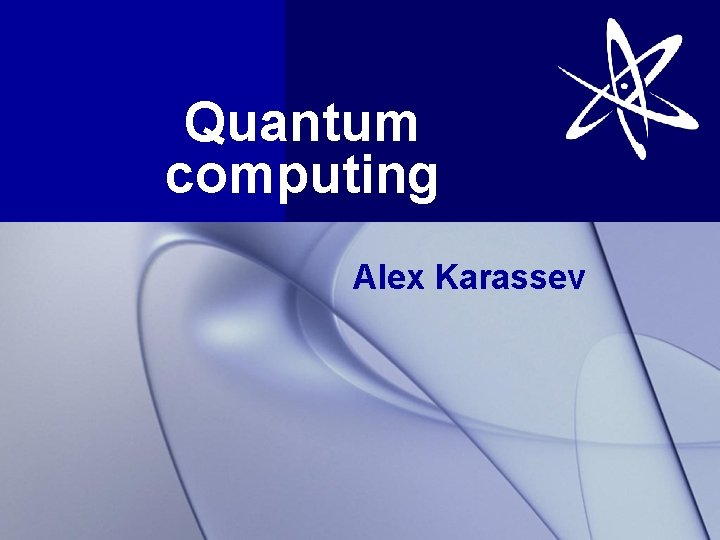Quantum computing Alex Karassev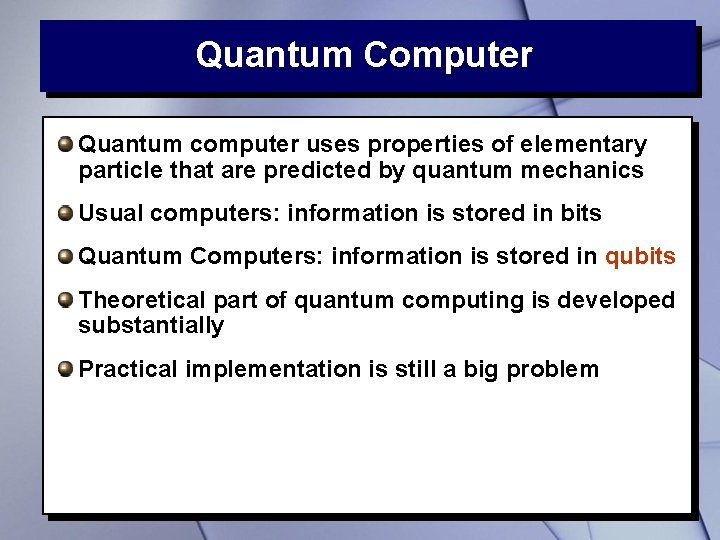Quantum Computer Quantum computer uses properties of elementary particle that are predicted by quantum mechanics Usual computers: information is stored in bits Quantum Computers: information is stored in qubits Theoretical part of quantum computing is developed substantially Practical implementation is still a big problem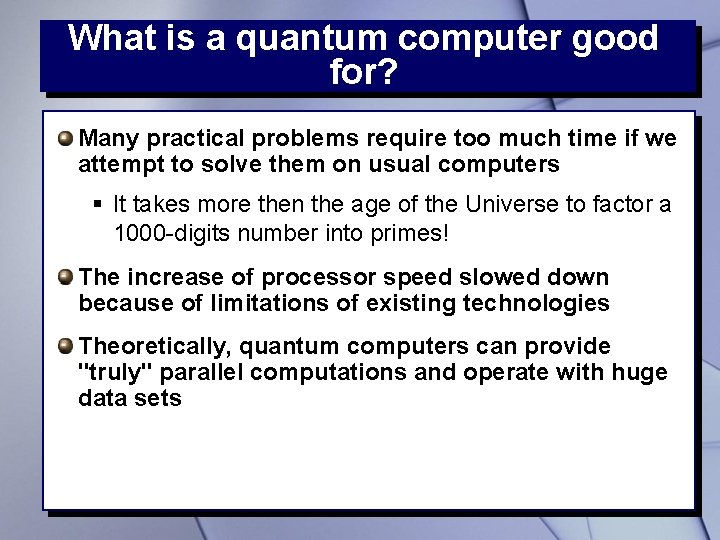What is a quantum computer good for? Many practical problems require too much time if we attempt to solve them on usual computers § It takes more then the age of the Universe to factor a 1000 -digits number into primes! The increase of processor speed slowed down because of limitations of existing technologies Theoretically, quantum computers can provide "truly" parallel computations and operate with huge data setsProbability questions How many times (in average) do we need to toss a coin to get a tail? How many times (in average) do we need to roll a die to get a six? Loaded die: alter a die so that the probability of getting 6 is 1/2.Quantum computers and probability When the quantum computer gives you the result of computation, this result is correct only with certain probability Quantum algorithms are designed to "shift" the probability towards correct result Running the same algorithm sufficiently many times you get the correct result with high probability, assuming that we can verify whether the result is correct or not The number of repetition is much smaller then for usual computersShort History 1970 -е: the beginning of quantum information theory 1980: Yuri Manin set forward the idea of quantum computations 1981: Richard Feynman proposed to use quantum computing to model quantum systems. He also describe theoretical model of quantum computer 1985: David Deutsch described first universal quantum computer 1994: Peter Shor developed the first algorithm for quantum computer (factorization into primes)Short History 1996: Lov Grover developed an algorithm for search in unsorted database 1998: the first quantum computers on two qubits, based on NMR (Oxford; IBM, MIT, Stanford) 2000: quantum computer on 7 qubits, based on NMR (Los-Alamos) 2001: 15 = 3 x 5 on 7 - qubit quantum comp. by IBM 2005 -2006: experiments with photons; quantum dots; fullerenes and nanotubes as "particle traps" 2007: D-Wave announced the creation of a quantum computer on 16 qubits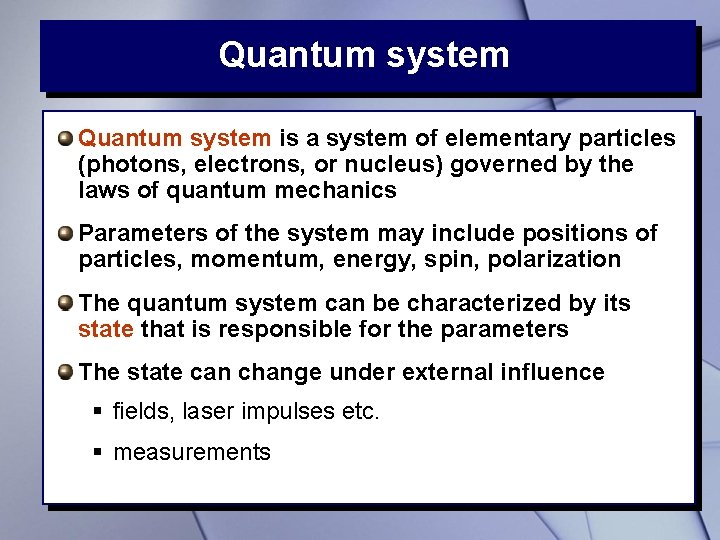Quantum system is a system of elementary particles (photons, electrons, or nucleus) governed by the laws of quantum mechanics Parameters of the system may include positions of particles, momentum, energy, spin, polarization The quantum system can be characterized by its state that is responsible for the parameters The state can change under external influence § fields, laser impulses etc. § measurements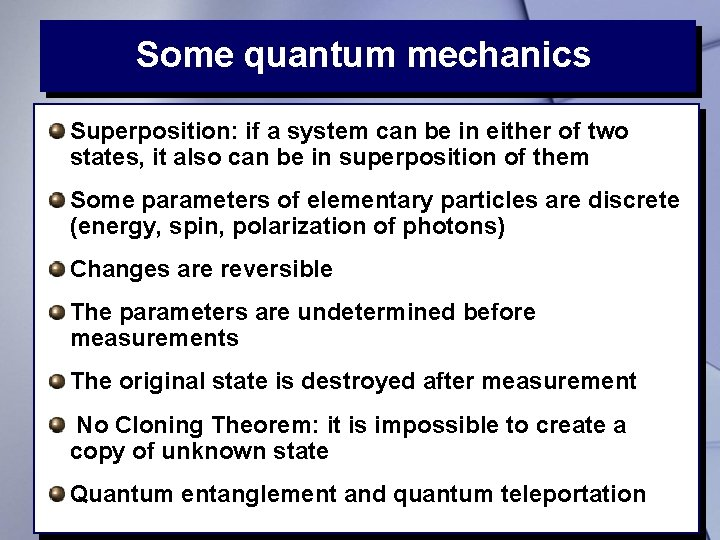Some quantum mechanics Superposition: if a system can be in either of two states, it also can be in superposition of them Some parameters of elementary particles are discrete (energy, spin, polarization of photons) Changes are reversible The parameters are undetermined before measurements The original state is destroyed after measurement No Cloning Theorem: it is impossible to create a copy of unknown state Quantum entanglement and quantum teleportation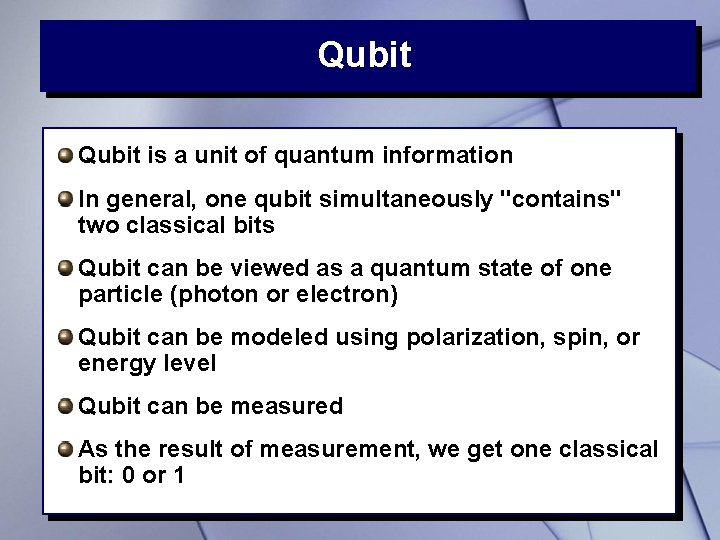Qubit is a unit of quantum information In general, one qubit simultaneously "contains" two classical bits Qubit can be viewed as a quantum state of one particle (photon or electron) Qubit can be modeled using polarization, spin, or energy level Qubit can be measured As the result of measurement, we get one classical bit: 0 or 1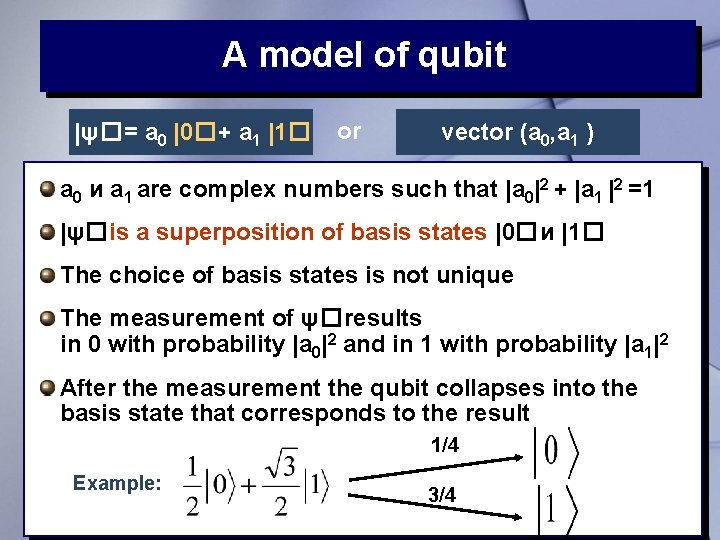A model of qubit |ψ�= a 0 |0�+ a 1 |1� or vector (a 0, a 1 ) a 0 и a 1 are complex numbers such that |a 0|2 + |a 1 |2 =1 |ψ�is a superposition of basis states |0�и |1� The choice of basis states is not unique The measurement of ψ�results in 0 with probability |a 0|2 and in 1 with probability |a 1|2 After the measurement the qubit collapses into the basis state that corresponds to the result 1/4 Example: 3/4Several qubits The system of n qubits "contain" 2 n classical bits (basis states) Thus the potential of a quantum computer grows exponentially We can measure individual qubits in the multi-qubit system § For example, in a two-qubit system we can measure the state of first or second qubit, or both The results of measurement are probabilistic After the measurement the system collapses in the corresponding state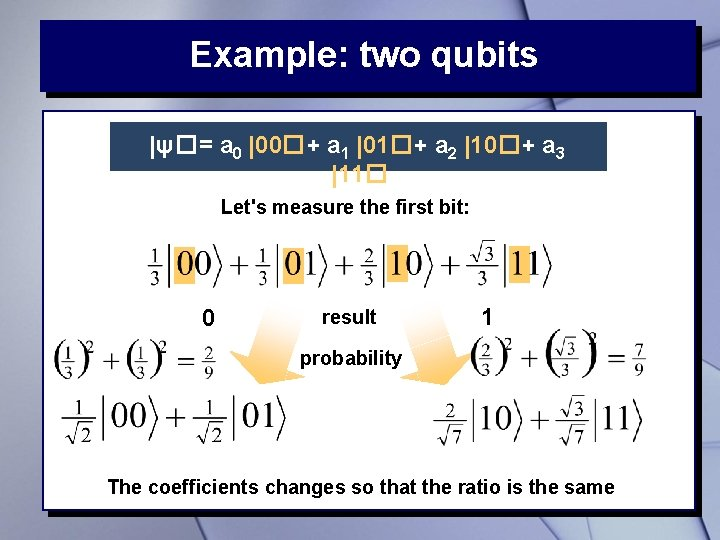Example: two qubits |ψ�= a 0 |00�+ a 1 |01�+ a 2 |10�+ a 3 |11� Let's measure the first bit: 0 result 1 probability The coefficients changes so that the ratio is the same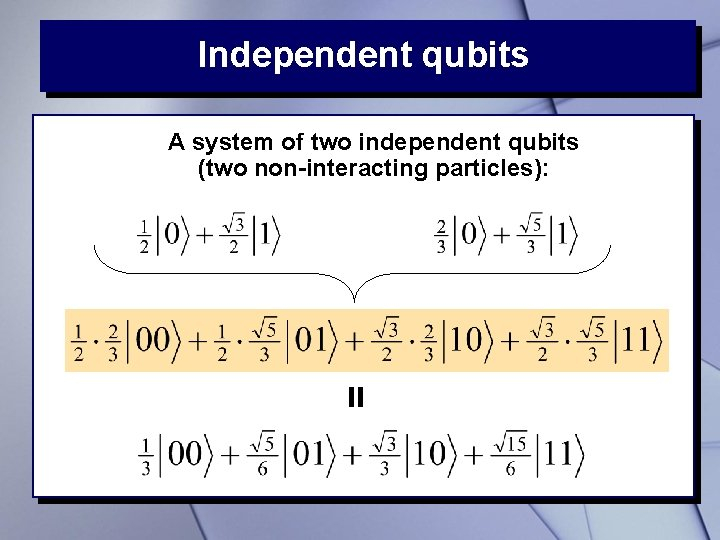Independent qubits = A system of two independent qubits (two non-interacting particles):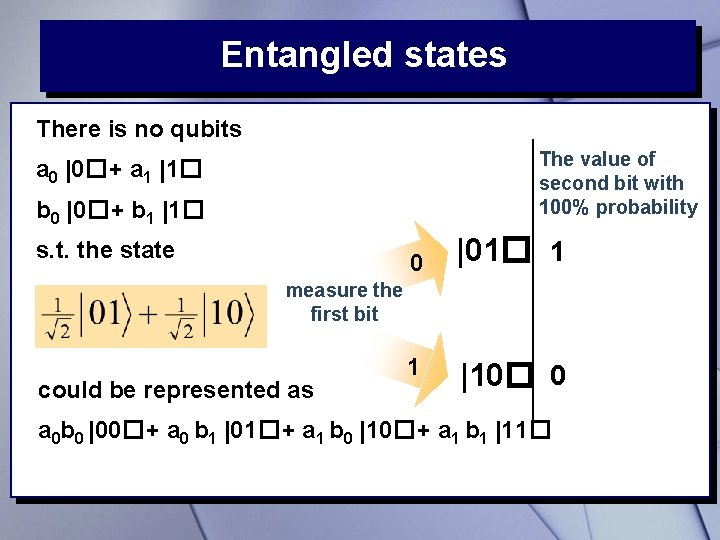Entangled states There is no qubits The value of second bit with 100% probability a 0 |0�+ a 1 |1� b 0 |0�+ b 1 |1� s. t. the state 0 |01� 1 measure the first bit could be represented as 1 |10� 0 a 0 b 0 |00�+ a 0 b 1 |01�+ a 1 b 0 |10�+ a 1 b 1 |11�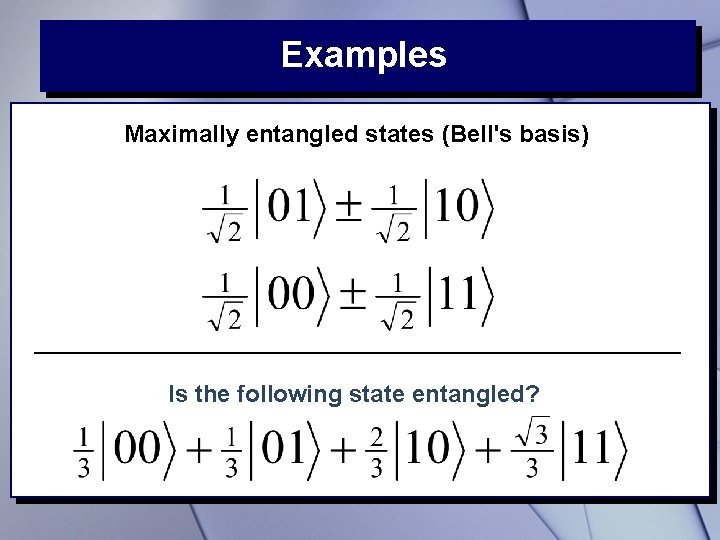Examples Maximally entangled states (Bell's basis) Is the following state entangled?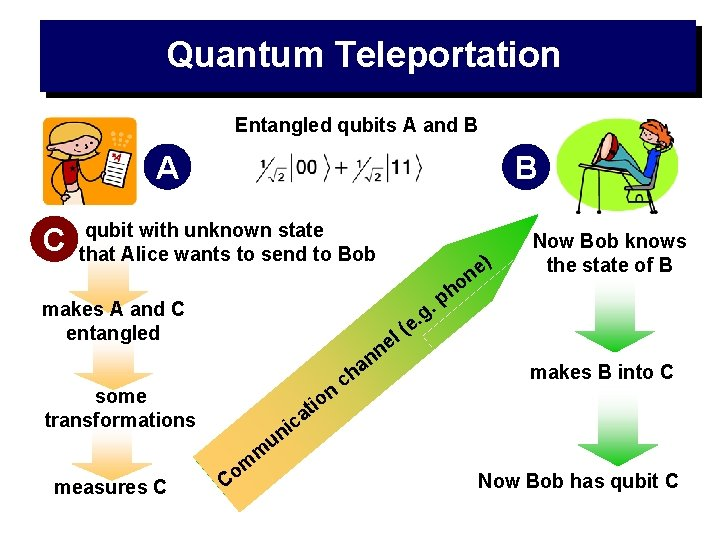Quantum Teleportation Entangled qubits A and B A C B qubit with unknown state that Alice wants to send to Bob . makes А and C entangled . g e l( e n n n it o some transformations measures C m m Co a h c ) e n o ph Now Bob knows the state of B makes B into C ca i un Now Bob has qubit C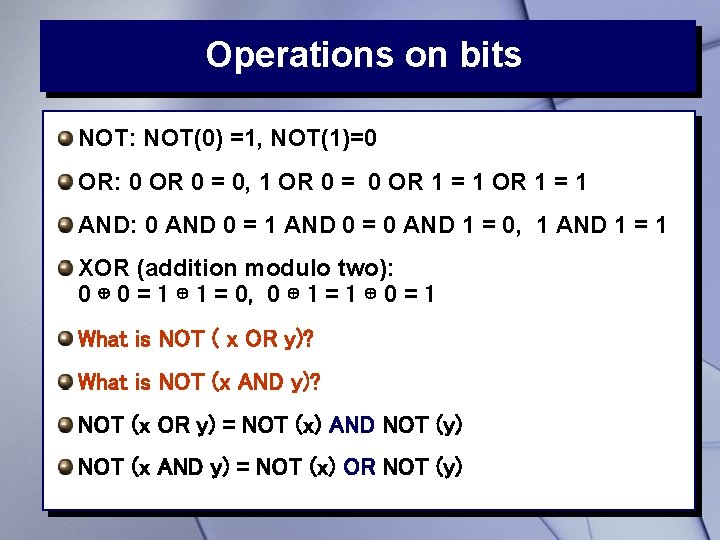Operations on bits NOT: NOT(0) =1, NOT(1)=0 OR: 0 OR 0 = 0, 1 OR 0 = 0 OR 1 = 1 AND: 0 AND 0 = 1 AND 0 = 0 AND 1 = 0, 1 AND 1 = 1 XOR (addition modulo two): 0 ⊕ 0 = 1 ⊕ 1 = 0, 0 ⊕ 1 = 1 ⊕ 0 = 1 What is NOT ( x OR y)? What is NOT (x AND y)? NOT (x OR y) = NOT (x) AND NOT (y) NOT (x AND y) = NOT (x) OR NOT (y)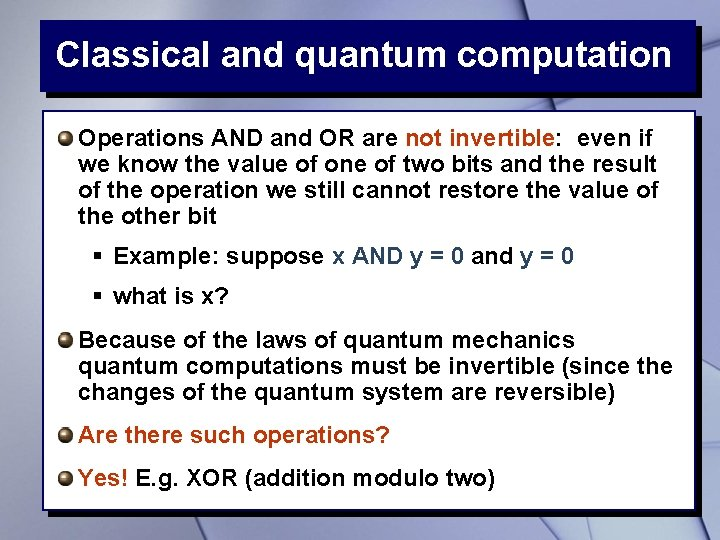Classical and quantum computation Operations AND and OR are not invertible: even if we know the value of one of two bits and the result of the operation we still cannot restore the value of the other bit § Example: suppose x AND y = 0 and y = 0 § what is x? Because of the laws of quantum mechanics quantum computations must be invertible (since the changes of the quantum system are reversible) Are there such operations? Yes! E. g. XOR (addition modulo two)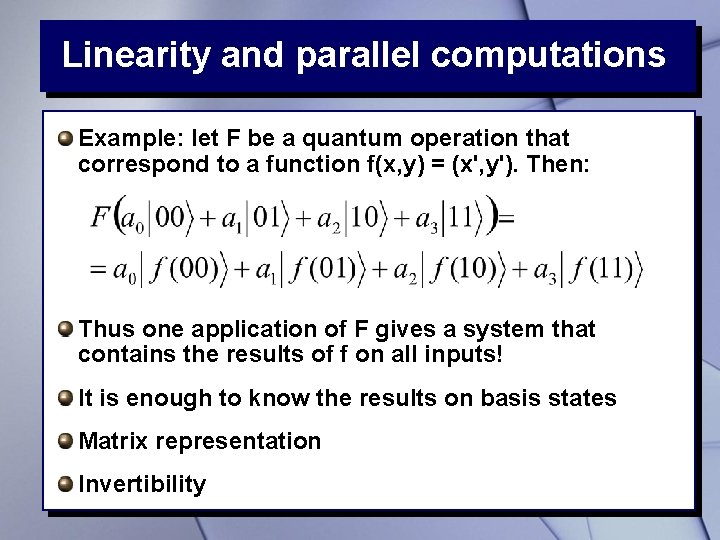Linearity and parallel computations Example: let F be a quantum operation that correspond to a function f(x, y) = (x', y'). Then: Thus one application of F gives a system that contains the results of f on all inputs! It is enough to know the results on basis states Matrix representation InvertibilitySome matrices… A matrix is a table of numbers, e. g. We can multiply matrices by vectors: Moreover, we even can multiply matrices!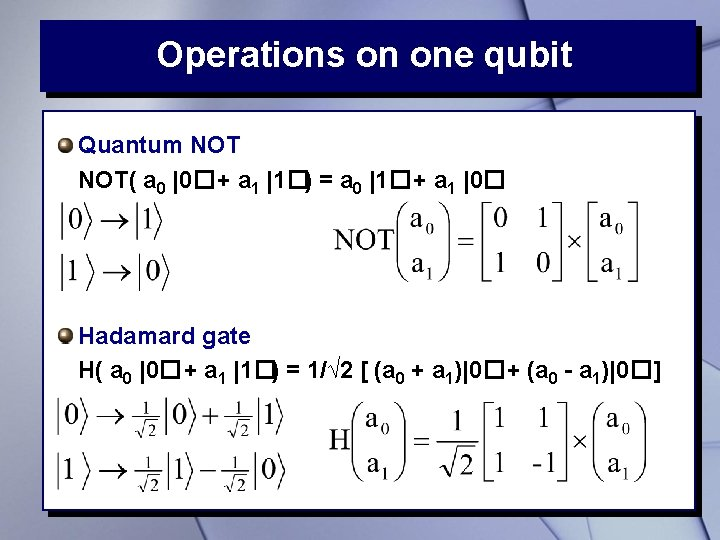Operations on one qubit Quantum NOT( a 0 |0�+ a 1 |1�) = a 0 |1�+ a 1 |0� Hadamard gate H( a 0 |0�+ a 1 |1�) = 1/√ 2 [ (a 0 + a 1)|0�+ (a 0 - a 1)|0�]Two qubits: controlled NOT (CNOT) CNOT (x, y) = (x, x XOR y)= (x, x⊕y) 0⊕ 0=1⊕ 1=0, 0⊕ 1=1⊕ 0=1 CNOT( a 0|00�+a 1|01�+a 2|10�+a 3|11�) = a 0|00�+a 1|01�+a 3|11�+a 2|10�How quantum computer works The routine § Initialization (e. g. all qubits are in state |0� § Quantum computations § Reading of the result (measurement) "Ideal" quantum computer: § must be universal (capable of performing arbitrary quantum operations with given precision) § must be scalable § must be able to exchange data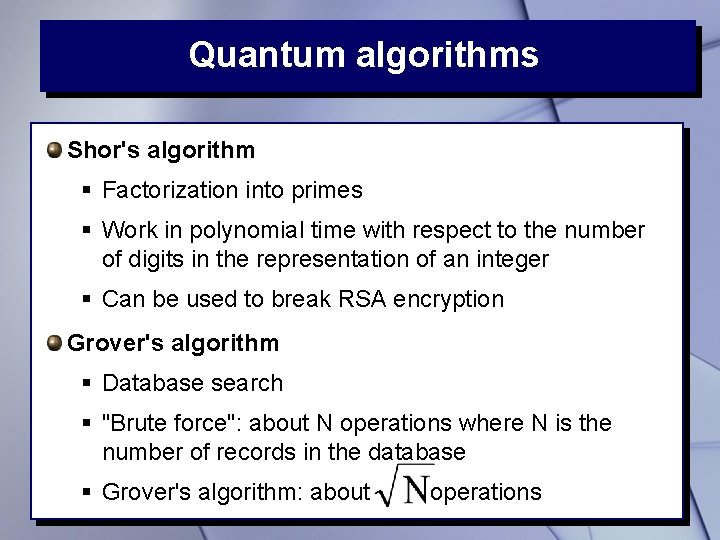Quantum algorithms Shor's algorithm § Factorization into primes § Work in polynomial time with respect to the number of digits in the representation of an integer § Can be used to break RSA encryption Grover's algorithm § Database search § "Brute force": about N operations where N is the number of records in the database § Grover's algorithm: about operationsProblems Decoherence § Quantum system is extremely sensitive to external environment, so it should be safely isolated § It is hard to achieve the decoherence time that is more than the algorithm running time Error correction (requires more qubits!) Physical implementation of computations New quantum algorithms to solve more problems Entangled states for data transfer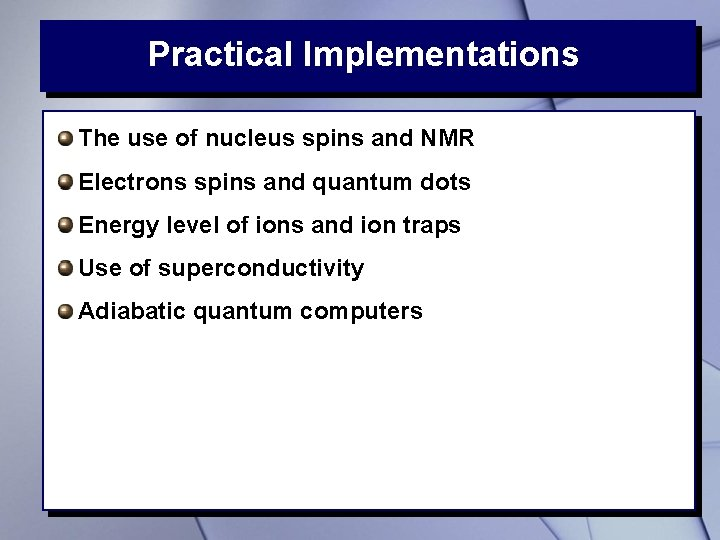Practical Implementations The use of nucleus spins and NMR Electrons spins and quantum dots Energy level of ions and ion traps Use of superconductivity Adiabatic quantum computersD-Wave: quantum computer Orion January 19, 2007: D-Wave Systems (Burnaby, British Columbia) announced a creation of a prototype of commercial quantum computer, called Orion According to D-Wave, adiabatic quantum computer Orion uses 16 qubits and can solve quite complex practical problems (e. g. search a database and solve Sudoku puzzle) Unfortunately, D-Wave did not disclose any technical details of their computer This caused a significant criticism among specialists Recently, the company received 17 millions investmentsHomework Is the following state entangled? What happens if we apply twice § negation? § Hadamard gate?Thank You! http: //www. nipissingu. ca/numeric http: //www. nipissingu. ca/faculty/alexandk/popular/ popular. html